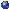## Events

##### Geometry And String TheoryCharlie Reid, Zoom: Quantization of the LQT theorem
Wednesday, September 15, 2021, 12:00pm - 01:00pm
Observables of GL(N+1) gauge theory restrict to observables of GL(N) gauge theory, so we may consider the inverse limit. The Loday-Quillen-Tsygan theorem gives a nice description of this inverse limit in terms of something called cyclic cohomology. A couple weeks ago, a paper came out by Ginot, Gwilliam, Hamilton, and Zeinlian which provides a "quantization" of the LQT theorem which in some sense describes quantum GL(N) gauge theory for large N. In this talk, we will first develop the algebra to state the LQT theorem and describe its role in field theory. Then, we will state the quantized theorem, and sketch how a very special case of this theorem recovers the theory of random matrices. This talk is meant to be another step in the direction of understanding the work of Costello, Li, and Paquette on AdS-CFT.Location: Zoom